Anúncio

# AMU - Physics - 2005

3 de Jan de 2012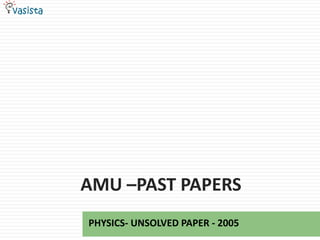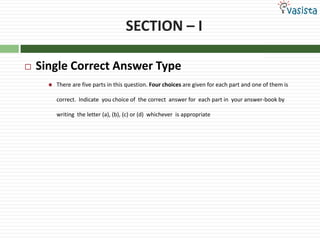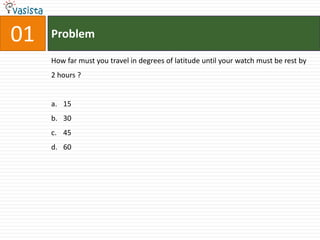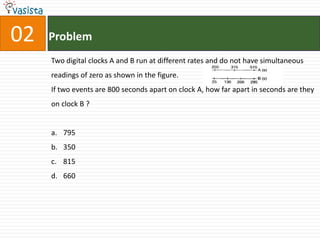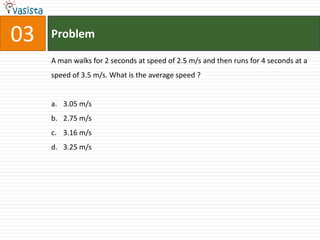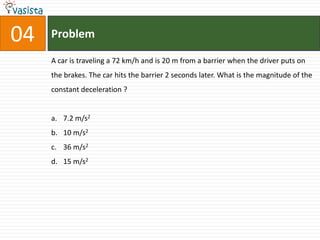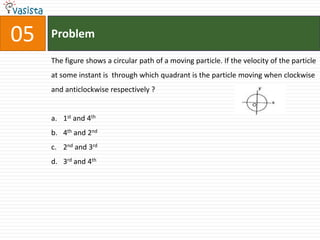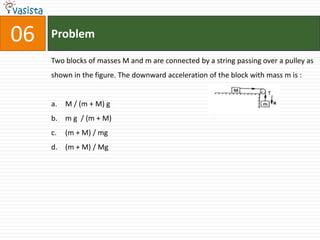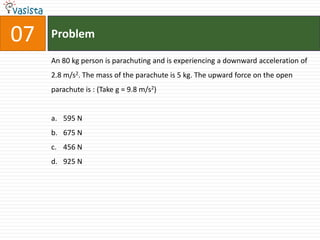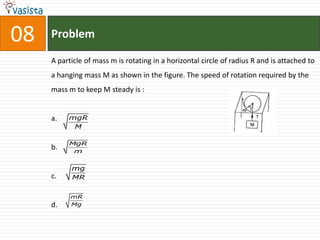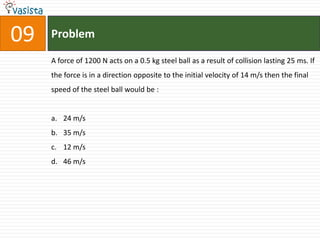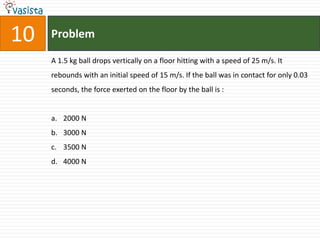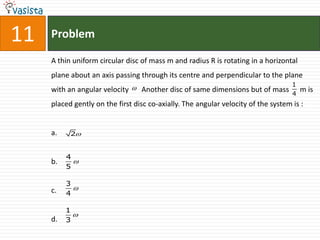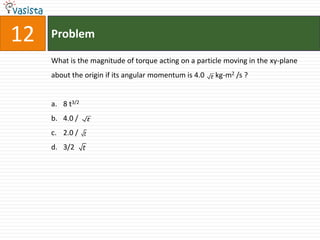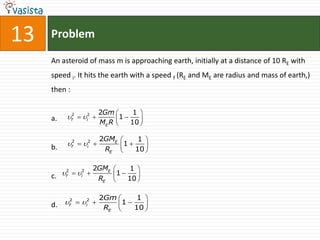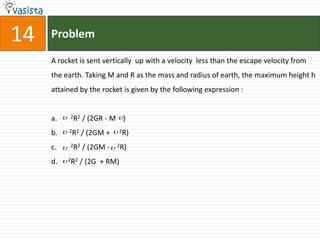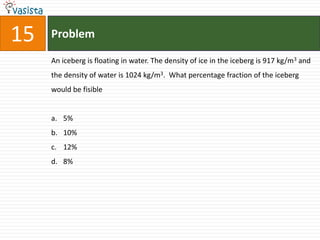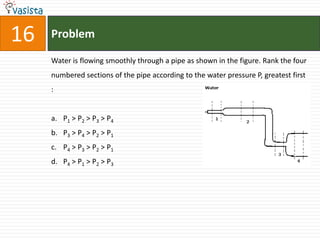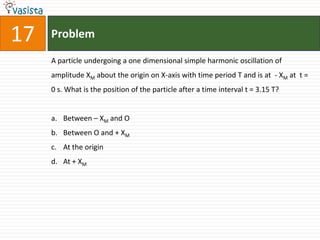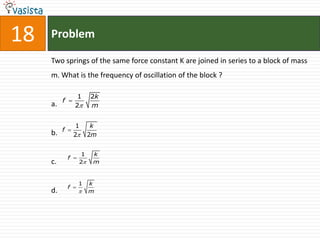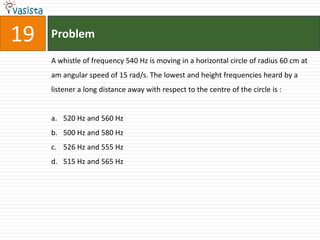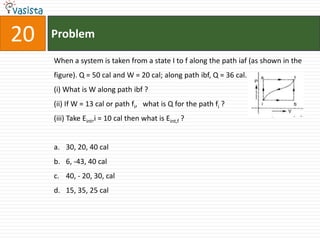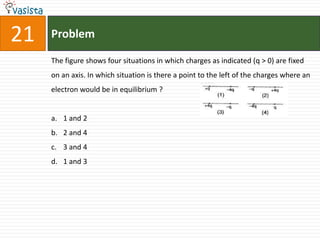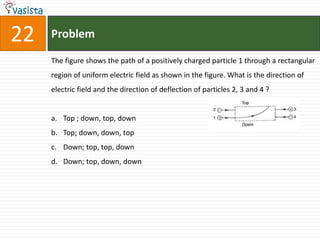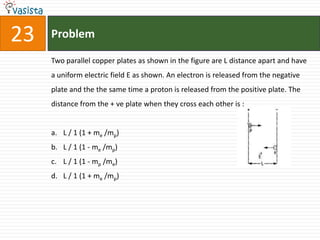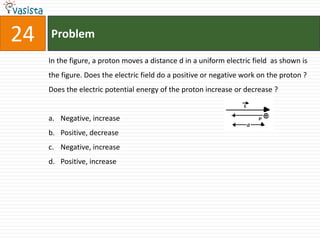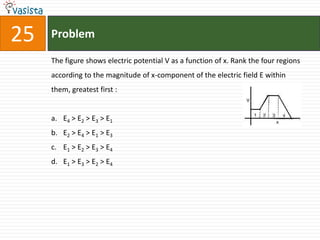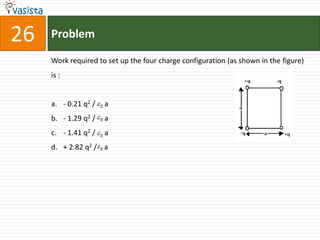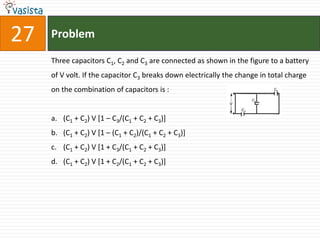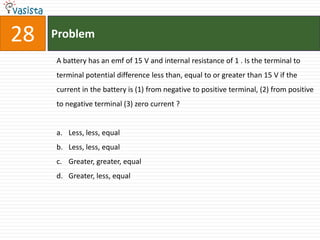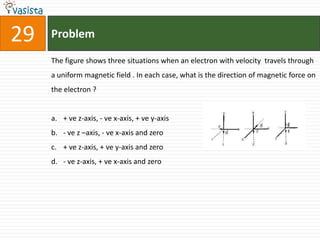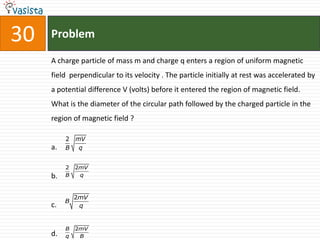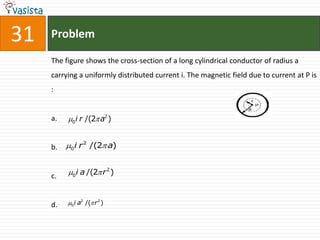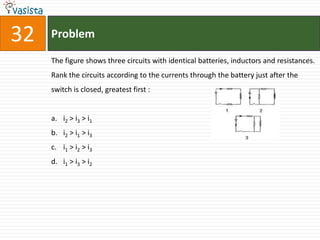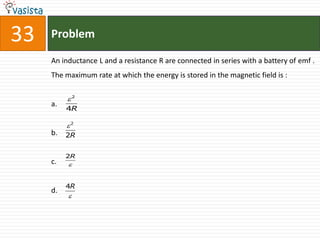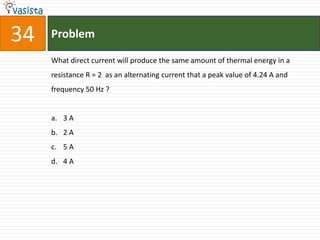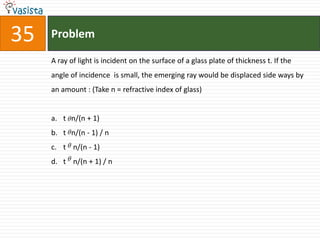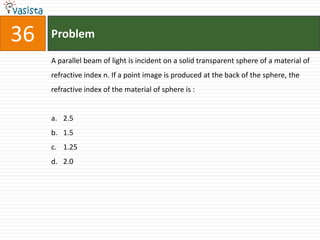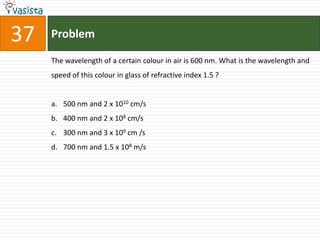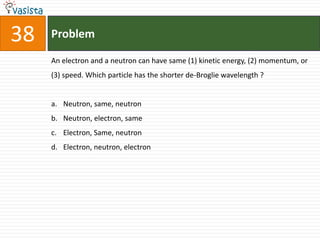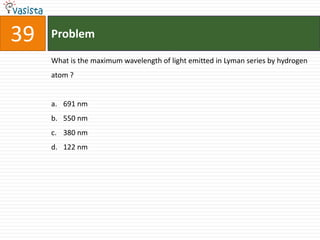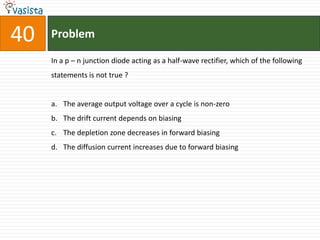1 de 43
Anúncio

### AMU - Physics - 2005

1. AMU –PAST PAPERS PHYSICS- UNSOLVED PAPER - 2005
2. SECTION – I  Single Correct Answer Type  There are five parts in this question. Four choices are given for each part and one of them is correct. Indicate you choice of the correct answer for each part in your answer-book by writing the letter (a), (b), (c) or (d) whichever is appropriate
3. 01 Problem How far must you travel in degrees of latitude until your watch must be rest by 2 hours ? a. 15 b. 30 c. 45 d. 60
4. 02 Problem Two digital clocks A and B run at different rates and do not have simultaneous readings of zero as shown in the figure. If two events are 800 seconds apart on clock A, how far apart in seconds are they on clock B ? a. 795 b. 350 c. 815 d. 660
5. 03 Problem A man walks for 2 seconds at speed of 2.5 m/s and then runs for 4 seconds at a speed of 3.5 m/s. What is the average speed ? a. 3.05 m/s b. 2.75 m/s c. 3.16 m/s d. 3.25 m/s
6. 04 Problem A car is traveling a 72 km/h and is 20 m from a barrier when the driver puts on the brakes. The car hits the barrier 2 seconds later. What is the magnitude of the constant deceleration ? a. 7.2 m/s2 b. 10 m/s2 c. 36 m/s2 d. 15 m/s2
7. 05 Problem The figure shows a circular path of a moving particle. If the velocity of the particle at some instant is through which quadrant is the particle moving when clockwise and anticlockwise respectively ? a. 1st and 4th b. 4th and 2nd c. 2nd and 3rd d. 3rd and 4th
8. 06 Problem Two blocks of masses M and m are connected by a string passing over a pulley as shown in the figure. The downward acceleration of the block with mass m is : a. M / (m + M) g b. m g / (m + M) c. (m + M) / mg d. (m + M) / Mg
9. 07 Problem An 80 kg person is parachuting and is experiencing a downward acceleration of 2.8 m/s2. The mass of the parachute is 5 kg. The upward force on the open parachute is : (Take g = 9.8 m/s2) a. 595 N b. 675 N c. 456 N d. 925 N
10. 08 Problem A particle of mass m is rotating in a horizontal circle of radius R and is attached to a hanging mass M as shown in the figure. The speed of rotation required by the mass m to keep M steady is : a. mgR M MgR b. m mg c. MR mR d. Mg
11. 09 Problem A force of 1200 N acts on a 0.5 kg steel ball as a result of collision lasting 25 ms. If the force is in a direction opposite to the initial velocity of 14 m/s then the final speed of the steel ball would be : a. 24 m/s b. 35 m/s c. 12 m/s d. 46 m/s
12. 10 Problem A 1.5 kg ball drops vertically on a floor hitting with a speed of 25 m/s. It rebounds with an initial speed of 15 m/s. If the ball was in contact for only 0.03 seconds, the force exerted on the floor by the ball is : a. 2000 N b. 3000 N c. 3500 N d. 4000 N
13. 11 Problem A thin uniform circular disc of mass m and radius R is rotating in a horizontal plane about an axis passing through its centre and perpendicular to the plane 1 with an angular velocity  Another disc of same dimensions but of mass 4 m is placed gently on the first disc co-axially. The angular velocity of the system is : a. 2 4 b.  5 3 c.  4 1  d. 3
14. 12 Problem What is the magnitude of torque acting on a particle moving in the xy-plane about the origin if its angular momentum is 4.0 t kg-m2 /s ? a. 8 t3/2 b. 4.0 / t c. 2.0 / t d. 3/2 t
15. 13 Problem An asteroid of mass m is approaching earth, initially at a distance of 10 RE with speed i. It hits the earth with a speed f (RE and ME are radius and mass of earth,) then : 2Gm  1  f2  i2  1 ME R  10  a.   2GME  1  f2  i2   1 b. RE  10   2GME  1  c. f2  i2  1  10  RE   2Gm  1  d. f  i  R 2 2  1 E  10  
16. 14 Problem A rocket is sent vertically up with a velocity less than the escape velocity from the earth. Taking M and R as the mass and radius of earth, the maximum height h attained by the rocket is given by the following expression : a.  2R2 / (2GR - M  ) b.  2R2 / (2GM +  2R) c.  2R2 / (2GM -  2R) d.  2R2 / (2G + RM)
17. 15 Problem An iceberg is floating in water. The density of ice in the iceberg is 917 kg/m3 and the density of water is 1024 kg/m3. What percentage fraction of the iceberg would be fisible a. 5% b. 10% c. 12% d. 8%
18. 16 Problem Water is flowing smoothly through a pipe as shown in the figure. Rank the four numbered sections of the pipe according to the water pressure P, greatest first : a. P1 > P2 > P3 > P4 b. P3 > P4 > P2 > P1 c. P4 > P3 > P2 > P1 d. P4 > P1 > P2 > P3
19. 17 Problem A particle undergoing a one dimensional simple harmonic oscillation of amplitude XM about the origin on X-axis with time period T and is at - XM at t = 0 s. What is the position of the particle after a time interval t = 3.15 T? a. Between – XM and O b. Between O and + XM c. At the origin d. At + XM
20. 18 Problem Two springs of the same force constant K are joined in series to a block of mass m. What is the frequency of oscillation of the block ? 1 2k f  a. 2 m 1 k f  b. 2 2m 1 k f  c. 2 m 1 k f  d.  m
21. 19 Problem A whistle of frequency 540 Hz is moving in a horizontal circle of radius 60 cm at am angular speed of 15 rad/s. The lowest and height frequencies heard by a listener a long distance away with respect to the centre of the circle is : a. 520 Hz and 560 Hz b. 500 Hz and 580 Hz c. 526 Hz and 555 Hz d. 515 Hz and 565 Hz
22. 20 Problem When a system is taken from a state I to f along the path iaf (as shown in the figure). Q = 50 cal and W = 20 cal; along path ibf, Q = 36 cal. (i) What is W along path ibf ? (ii) If W = 13 cal or path fi, what is Q for the path fi ? (iii) Take Eint,i = 10 cal then what is Eint,f ? a. 30, 20, 40 cal b. 6, -43, 40 cal c. 40, - 20, 30, cal d. 15, 35, 25 cal
23. 21 Problem The figure shows four situations in which charges as indicated (q > 0) are fixed on an axis. In which situation is there a point to the left of the charges where an electron would be in equilibrium ? a. 1 and 2 b. 2 and 4 c. 3 and 4 d. 1 and 3
24. 22 Problem The figure shows the path of a positively charged particle 1 through a rectangular region of uniform electric field as shown in the figure. What is the direction of electric field and the direction of deflection of particles 2, 3 and 4 ? a. Top ; down, top, down b. Top; down, down, top c. Down; top, top, down d. Down; top, down, down
25. 23 Problem Two parallel copper plates as shown in the figure are L distance apart and have a uniform electric field E as shown. An electron is released from the negative plate and the the same time a proton is released from the positive plate. The distance from the + ve plate when they cross each other is : a. L / 1 (1 + me /mp) b. L / 1 (1 - me /mp) c. L / 1 (1 - mp /me) d. L / 1 (1 + me /mp)
26. 24 Problem In the figure, a proton moves a distance d in a uniform electric field as shown is the figure. Does the electric field do a positive or negative work on the proton ? Does the electric potential energy of the proton increase or decrease ? a. Negative, increase b. Positive, decrease c. Negative, increase d. Positive, increase
27. 25 Problem The figure shows electric potential V as a function of x. Rank the four regions according to the magnitude of x-component of the electric field E within them, greatest first : a. E4 > E2 > E3 > E1 b. E2 > E4 > E1 > E3 c. E1 > E2 > E3 > E4 d. E1 > E3 > E2 > E4
28. 26 Problem Work required to set up the four charge configuration (as shown in the figure) is : a. - 0.21 q2 /  0 a b. - 1.29 q2 /  0 a c. - 1.41 q2 /  0 a d. + 2.82 q2 / 0 a
29. 27 Problem Three capacitors C1, C2 and C3 are connected as shown in the figure to a battery of V volt. If the capacitor C3 breaks down electrically the change in total charge on the combination of capacitors is : a. (C1 + C2) V [1 – C3/(C1 + C2 + C3)] b. (C1 + C2) V [1 – (C1 + C2)/(C1 + C2 + C3)] c. (C1 + C2) V [1 + C3/(C1 + C2 + C3)] d. (C1 + C2) V [1 + C2/(C1 + C2 + C3)]
30. 28 Problem A battery has an emf of 15 V and internal resistance of 1 . Is the terminal to terminal potential difference less than, equal to or greater than 15 V if the current in the battery is (1) from negative to positive terminal, (2) from positive to negative terminal (3) zero current ? a. Less, less, equal b. Less, less, equal c. Greater, greater, equal d. Greater, less, equal
31. 29 Problem The figure shows three situations when an electron with velocity travels through a uniform magnetic field . In each case, what is the direction of magnetic force on the electron ? a. + ve z-axis, - ve x-axis, + ve y-axis b. - ve z –axis, - ve x-axis and zero c. + ve z-axis, + ve y-axis and zero d. - ve z-axis, + ve x-axis and zero
32. 30 Problem A charge particle of mass m and charge q enters a region of uniform magnetic field perpendicular to its velocity . The particle initially at rest was accelerated by a potential difference V (volts) before it entered the region of magnetic field. What is the diameter of the circular path followed by the charged particle in the region of magnetic field ? 2 mV a. B q 2 2mV b. B q 2mV B c. q B 2mV d. q B
33. 31 Problem The figure shows the cross-section of a long cylindrical conductor of radius a carrying a uniformly distributed current i. The magnetic field due to current at P is : a. 0 i r /(2 a2 ) b. 0 i r 2 /(2 a) c. 0 i a /(2 r 2 ) d. 0 i a2 /( r 2 )
34. 32 Problem The figure shows three circuits with identical batteries, inductors and resistances. Rank the circuits according to the currents through the battery just after the switch is closed, greatest first : a. i2 > i3 > i1 b. i2 > i1 > i3 c. i1 > i2 > i3 d. i1 > i3 > i2
35. 33 Problem An inductance L and a resistance R are connected in series with a battery of emf . The maximum rate at which the energy is stored in the magnetic field is : 2 a. 4R 2 b. 2R 2R c.  4R d. 
36. 34 Problem What direct current will produce the same amount of thermal energy in a resistance R = 2 as an alternating current that a peak value of 4.24 A and frequency 50 Hz ? a. 3 A b. 2 A c. 5 A d. 4 A
37. 35 Problem A ray of light is incident on the surface of a glass plate of thickness t. If the angle of incidence is small, the emerging ray would be displaced side ways by an amount : (Take n = refractive index of glass) a. t  n/(n + 1) b. t  n/(n - 1) / n c. t  n/(n - 1) d. t  n/(n + 1) / n
38. 36 Problem A parallel beam of light is incident on a solid transparent sphere of a material of refractive index n. If a point image is produced at the back of the sphere, the refractive index of the material of sphere is : a. 2.5 b. 1.5 c. 1.25 d. 2.0
39. 37 Problem The wavelength of a certain colour in air is 600 nm. What is the wavelength and speed of this colour in glass of refractive index 1.5 ? a. 500 nm and 2 x 1010 cm/s b. 400 nm and 2 x 108 cm/s c. 300 nm and 3 x 109 cm /s d. 700 nm and 1.5 x 108 m/s
40. 38 Problem An electron and a neutron can have same (1) kinetic energy, (2) momentum, or (3) speed. Which particle has the shorter de-Broglie wavelength ? a. Neutron, same, neutron b. Neutron, electron, same c. Electron, Same, neutron d. Electron, neutron, electron
41. 39 Problem What is the maximum wavelength of light emitted in Lyman series by hydrogen atom ? a. 691 nm b. 550 nm c. 380 nm d. 122 nm
42. 40 Problem In a p – n junction diode acting as a half-wave rectifier, which of the following statements is not true ? a. The average output voltage over a cycle is non-zero b. The drift current depends on biasing c. The depletion zone decreases in forward biasing d. The diffusion current increases due to forward biasing
43. FOR SOLUTIONS VISIT WWW.VASISTA.NET
Anúncio